## Main

The integer quantum Hall effect, discovered nearly thirty years ago1, has recently experienced a strong revival driven by milestone experiments towards quantum information with edge states7,11,12. Beyond Hall currents, new phenomena have emerged that were unexpected within the free one-dimensional chiral fermions (1DCFs) model. The ongoing debate triggered by electronic Mach–Zehnder interferometer experiments7,13,14,15 vividly illustrates the gaps in our understanding. Coulomb interaction is seen as the key ingredient. In addition to its most striking repercussion, the fractional quantum Hall effect16, the edge reconstruction turns out to have deep implications on edge excitations. This phenomenon results from the competition between Coulomb interaction that tends to spread the electronic fluid, and the confinement potential: as the latter gets smoother, the non-interacting edge becomes unstable17. Theory predicts new branches of gapless electronic excitations in reconstructed edges10,18, which breaks the mapping of an edge channel onto 1DCFs and, possibly, the promising quantum optics analogy. For most edges realized in semiconductor heterojunctions (except by cleaved edge overgrowth19), edge reconstruction results in wide compressible edge channels separated by narrow incompressible strips9 and the new excited states are overall neutral internal charge oscillations across the edge channels’ width10.

In practice, the predicted extra neutral modes are transparent to Hall currents. More surprisingly, a linear IV characteristic is frequently observed for tunnel contacts (different behaviours were also reported, for example, ref. 20), whereas a nonlinear characteristic is predicted21,22,23. This contradiction is resolved by assuming ad-hoc that only rigid displacements of compressible edge channels are excited by tunnel events, and not internal excitations10,21,22,24. The rigid displacement model arguably relies on the overriding strength of Coulomb interaction that tends to orthogonalize bare tunnelling electrons and correlated electronic fluids24. However, the above argument does not hold at arbitrary transmission probabilities, where several electron processes occur. Therefore, the role of predicted internal excitations has to be determined experimentally. The present work provides such a test. An edge channel is driven out-of-equilibrium with a quantum point contact (QPC) of arbitrary transmission, possibly exciting internal modes. A short distance away, the resulting energy distribution f(E) is measured with a tunnel-coupled quantum dot expected to probe only rigid displacement excitations, hereafter called quasiparticles. Consequently, the amount of energy injected into internal modes at the QPC would appear as an energy loss in f(E).

Measurements of the energy distribution in mesoscopic devices were first carried out in 1997 on metallic circuits using a superconducting tunnel probe25. In two-dimensional electron-gas systems, non-Fermi energy distributions could not be measured because transferring the techniques developed for metal circuits is technically challenging (although hot electrons have been detected, for example, ref. 26). Regarding the quantum Hall regime, the state of the art is the very recent qualitative probe of heating27. Here, we demonstrate that f(E) can be fully extracted from the tunnel current across a quantum dot. In the sequential tunnelling regime, the discrete electronic levels in a quantum dot behave as energy filters28, as previously demonstrated with double quantum dots29. Assuming a single active quantum-dot level of energy Elev, and ignoring the energy dependence of tunnel rates and tunnelling density of states in the electrodes, the quantum-dot current reads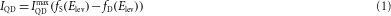where the subscript S (D) refers to the source (drain) electrode; fS,D are the corresponding energy distributions and IQDmax is the maximum quantum-dot current. In practice, fS,D are obtained separately by applying a large enough source–drain voltage (Fig. 1a,b) and the probed energy Elev=E0e ηGVG is swept using a capacitively coupled gate biased at VG, with ηG being the gate voltage-to-energy lever arm and E0 an offset. Raw data IQD/ VG measured by lock-in techniques are proportional to fD,S(E)/ E.

A tunable non-Fermi energy distribution is generated in an edge channel with a voltage-biased QPC. Similar set-ups were used previously to create imbalanced electron populations between co-propagating edge channels30, each characterized by a cold Fermi distribution. Only in a very recent experiment27 was an edge channel heated up. Beyond heating, f(E) is here controllably tuned out-of-equilibrium. Let us consider one edge channel and assume it can be mapped onto non-interacting 1DCFs. According to the scattering approach5, the energy distribution at the output of a QPC of transmission τ is a tunable double step (Fig. 1c, left inset)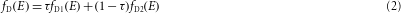where fD1 (fD2) is the equilibrium Fermi distribution function in the partially transmitted (reflected) incoming edge channel of electrochemical potential shifted by e VD1 (e VD2). In the presence of edge reconstruction, the above energy distribution applies to the quasiparticles if internal modes are not excited at the QPC. On the other hand, if internal modes are excited, there are no theoretical predictions because a QPC is very difficult to treat non-perturbatively in their natural bosonic formalism.

The sample shown in Fig. 1c was tailored in a two-dimensional electron gas realized in a GaAs/Ga(Al)As heterojunction, set to filling factor two and measured in a dilution refrigerator of base temperature 30 mK. The experiment detailed here focuses on the outer edge channel represented as a white line. The inner edge channel (not shown) is fully reflected by the QPC and the quantum dot. We checked that charge tunnelling between edge channels is negligible along the 0.8 μm propagation length from the QPC to the quantum dot.

We first carry out a standard nonlinear quantum-dot characterization28 (Fig. 2, top left inset). The two large signal stripes are frontiers of consecutive Coulomb diamonds and are accounted for by a single active quantum-dot level. Small contributions of three extra levels of relative energies {−95,30,130} μeV are also visible. The lever arm extracted from the stripes’ slopes is ηG0.052±9%.

Then, we test the spectroscopy with known Fermi functions by measuring IQD/ VG(VG) at VD1=VD2=−88 μV for several temperatures (Fig. 2, bottom right inset). By fitting these data with equation (1) using Fermi functions, we extract a fit temperature scaled by the lever arm Tfit/ηG. The value ηG=0.057, compatible with the nonlinear quantum-dot characterization, is found to reproduce best the mixing-chamber temperature T with Tfit. The drain and source fit temperatures are shown in Fig. 2, together with Tfit obtained using the standard procedure28 from IQD/ VD(VG) at VD0. We find deviations mostly within ±10% (dashed lines in Fig. 2) except for a saturation at Tfit≈50 mK possibly owing to a higher electronic temperature. In the following, we use ηG=0.057 obtained here in the same experimental configuration as to measure unknown f(E)s.

Electrons are now driven out-of-equilibrium in the drain outer edge channel. In the following, the electrode D2 and the inner drain edge channel are voltage biased at VD2=−88 μV and the source edge channels are emitted by a cold ground.

First, the bias voltage across the QPC is set to δ VDVD1VD2=36 μV and its conductance GQPC=τ e2/h is tuned by applying VQPC to the bottom left gate in Fig. 1c (see Fig. 3a). Note that at 30 mK, we find the transmission τ is constant within 2% with the QPC voltage bias below 36 μV. Typical sweeps IQD/ VG(VG) and the corresponding fD(E) are shown in Fig. 3b and e, respectively. The quantum-dot drain negative contribution transforms from a single dip at τ={0,1} into two dips separated by a fixed gate voltage and with relative weights that evolve monotonously with τ]0,1[. The solid lines are fits with equation (2) using for fD1,D2 two Fermi functions shifted by a fixed energy and weighted by the factors τfit and 1−τfit. The values of τfit are found to deviate by less than 0.03 from the measured transmission τ (Fig. 3c), in accurate agreement with the free 1DCF model. The plus symbols in Fig. 3 correspond to data obtained in a second cooldown.Figure 3: Spectroscopy of an edge channel tuned out-of-equilibrium with the conductance of a QPC.

In a second step, the QPC transmission is fixed to τ≈0.5 and the bias voltage δ VD is changed. Typical raw data are shown in Fig. 4a. These were obtained in a third cooldown with a quantum dot renewed by the thermal cycle showing no signs of extra quantum-dot levels in the probed energy range. The single dip in the quantum-dot drain contribution (bright) at δ VD=0 splits into two similar dips that are separated by a gate-voltage difference proportional to δ VD. In contrast, the quantum-dot source peak (dark) is mostly unchanged but slowly drifts parallel to one quantum-dot drain dip owing to the capacitive coupling between the drain and the quantum dot. In the first cooldown, VD1 was kept within [−106,−34] μV to minimize complications related to extra quantum-dot levels (lower bound) and to ensure well-separated source and drain contributions (upper bound). The symbols in Fig. 4b and e are, respectively, data and extracted fD(E) for the quantum-dot drain contribution at δ VD={−18,0,18,27,36,45,54} μV and τ=0.58. The solid lines in Fig. 4b are fits with equation (2) using the measured τ and for fD1,D2 two Fermi functions shifted in energy by the fit parameter −e ηGδ VG. The resulting ηGδ VG are plotted as symbols versus δ VD in Fig. 4c. Those obtained in the third cooldown are shown as purple star symbols using the renewed lever arm ηG=0.062. We find ηGδ VGδ VD as expected from the non-interacting 1DCF model. Deviations are always smaller than 8 μV (5 μV) for the first (third) cooldown, a reasonable agreement regarding uncertainties in ηG of ±10% (±5%).Figure 4: Spectroscopy of an edge channel tuned out-of-equilibrium with the voltage across a QPC.

In the two experiments above, we found the measured quasiparticle f(E)s verify predictions of the scattering approach. To establish the QPC/beam-splitter analogy one also needs to demonstrate that internal edge-channel modes are not excited. A direct test consists of extracting the quasiparticle heat current JEqp from the data, and comparing it with the full edge-excitations’ heat current JE obtained from power-balance considerations (see Supplementary Information for details):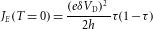The cancellation v ν=1/h of velocity (v) and density of states per unit length and energy (ν) that applies to the 1DCF quasiparticles permits us to obtain JEqp from the measured f(E) without any sample-specific parameters: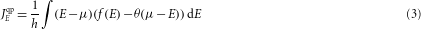where μ is the electrochemical potential and θ(E) is the step function. Consequently, we measure quantitatively the quasiparticle heat current. The result of this procedure is shown as symbols in Figs 3d and 4d using the generalized non-equilibrium temperature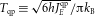together with the prediction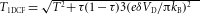if none of the injected power is carried on by internal modes (solid lines). We find a good agreement TqpT1DCF without fitting parameters and essentially in or close to error bars. Hence, within our experimental accuracy, the propagative internal modes do not contribute to heat transport and therefore are not excited. Note that the relatively small observed deviations are cooldown dependent, which suggests that the quantum-dot detector is responsible for these deviations. Indeed, the data can be more accurately accounted for including a second active quantum-dot level (see Supplementary Information). Last, preliminary data show a significant energy redistribution with the inner edge channel for propagations longer than 2 μm in the probed energy range. Therefore, the observed small discrepancies could also result from the finite 0.8 μm propagation length.

Overall, we demonstrate that QPCs in the quantum Hall regime are tunable electrical beam splitters for one-dimensional fermions, that is, rigid edge-channel displacements, (1) by comparing the energy distribution at a QPC output with predictions of the scattering approach5, and (2) by showing that internal edge-channel modes are not excited. This does not only rule out non-ideal QPC behaviours to explain the surprising phenomena observed on electronic Mach–Zehnder interferometers7,13,14,15, it also establishes a solid ground for future quantum information applications with edge states. Finally, an essential part of this work is the demonstration of a new technique to measure the fundamental energy-distribution function. It makes f(E) accessible for most systems where quantum dots can be realized. We expect it will trigger many new experiments dealing with heat transport, out-of-equilibrium physics and quantum decoherence.